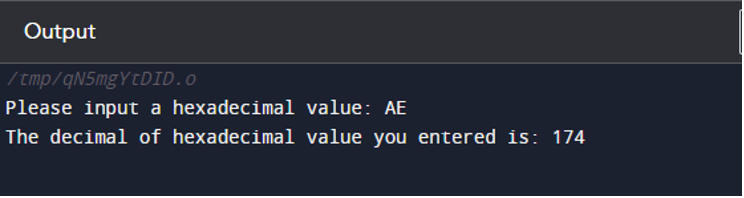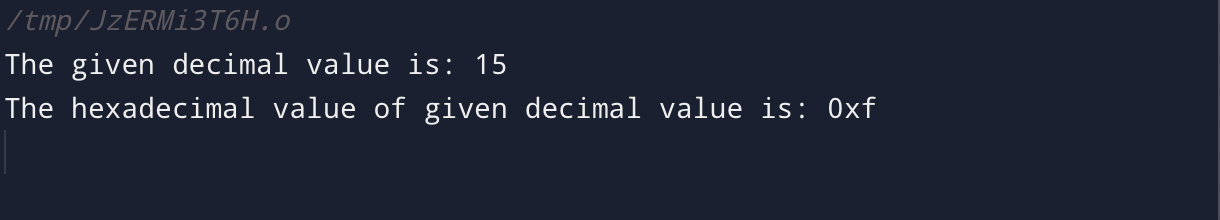| by Arround The Web | No comments

Hexadecimal integers are frequently used in computing for expressing binary values in a more comprehensible way. Base-16 numbers known as hexadecimal numbers are widely used in a variety of programming languages, including C. We will go through how to read and write hexadecimal values and also elaborate on it in using the C program in this tutorial for your better understanding.

## What is Hexadecimal Value in C?

A hexadecimal value in the C programming language is a base-16 formatted number. Binary values are frequently represented in a more understandable manner using hexadecimal numbers.

Hexadecimal numbers are made up of the numerals 0 through 9 and the letters A through F (or a–f), where each digit stands for a number between 0 and 15. The numbers 10 to 15 are represented by the letters A to F.

Hexadecimal numbers are represented in C by a prefix “0x” or “0X” that precedes the digits. For instance, the following syntax can be used to assign an integer variable the hexadecimal value 0x3F:

int hexdecimal_Value = 0x3F;

Additionally, we may output a hexadecimal value on our screen by using the printf() function and the “%x” format specifier:

int hexdecimal_Value = 123;
printf("The hexadecimal value is: 0x%x", hexdecimal_Value);

This will output “The hexadecimal value is: 0x7b” on the screen. Now, we will see the C program implementation of the read and write method to show hexadecimal value on the prompt.

Using the “%x” format specifier and the scanf() method, we may read hexadecimal data in C. It instructs scanf() to interpret the value entered into a hexadecimal number using this format specifier.

The following is an illustration of a program that reads a user-inputted hexadecimal value and displays it on the screen:

#include <stdio.h>

int main() {
int value_entered;
scanf("%x", &value_entered);
printf("The decimal of hexadecimal value you entered is: %d\n", value_entered);
return 0;
}

We initially declare an int variable named “value_entered” in this program. We then use the printf() method to ask the user to input a hexadecimal value. The input is then read as a hexadecimal number using the scanf() function and the “%x” format specifier, and it is then saved in the “value_entered” variable.

Finally, we printed the value provided by the user using the printf() method. The decimal value of the hexadecimal value is printed with the “%d” format specifier.

When you execute the program, the following output will show on the display:## How to Write Hexadecimal Value in C?

We can use the printf() function and the “%x” format specifier in C to write hexadecimal values. Below is an example that writes a value in hexadecimal in C language:

#include <stdio.h>

int main() {
int decimal_value = 15;
printf("The given decimal value is: %d\n", decimal_value);
printf("The hexadecimal value of given decimal value is: 0x%x\n", decimal_value);
return 0;
}

In this example code, the integer variable “decimal_value” is initially declared, and its value is set to 15 in decimal form.

The decimal number of the variable “decimal_value” is then printed utilizing the “%d” format specifier and the printf() function.

The contents of the variable “decimal_value” is then printed on the screen using the printf() function once more. This time, we use the “%x” format specifier along with the “0x” prefix to show that the value of the variable is in hexadecimal format.

When you run this script, the screen will first display the decimal value 15, then the hexadecimal value as shown below:## Conclusion

We covered ways to read and write hexadecimal numbers in C in this guide. The prevalence of hexadecimal numerals in numerous programming languages makes them a crucial component of computer programming. Hexadecimal numbers are simple to read and write in C, using the “%x” format specifier, the scanf(), and printf() functions.

Source: linuxhint.com